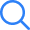# How Many Chapters Are There In Maths Class 7 NCERT?

15 chapters are there in NCERT Class 7 Maths & developing a strong grip on each subject is essential for the students. To completely finish the given chapters students can take help of the CBSE Class 7 Syllabus to know what chapters and topics they will be required to cover.

All these 15 chapters are very important from the annual exam perspective but there are a few chapters that have a bit higher weightage in class 7 maths exam.

The important chapters of class 7 maths from the exam perspective are given below.

## Which chapters are important In class 7 Maths from an exam's perspective?

As per the syllabus where the weightage table is given, we can see that chapters Fractions and Decimals, Data Handling, Simple Equations and Lines and Angles have the highest weightage due to which these are more important.

Integers, Mensuration, Comparing Quantities and Geometry are also very important chapters for Class 7 Maths.

To learn these chapters, a student will have to go through various definitions & examples then they should move to the actual exercises.

## How To Cover 15 Chapter of Class 7 Maths In Less Time?

Completing 15 chapters of class 7 maths is not a very tough task to do. It is quite interesting and easy to do. In order to complete the 15 chapters you just need to go through the whole syllabus and know the subtopics you have to study.

After that you need to stick to the NCERT Maths books of class 7 and solve its example questions and all the exercises. In case you face difficulty you can choose to use the NCERT Solutions for Class 7 Maths.

Apart from this, you can even refer to the revision notes to comprehend the topics in a much easier way. Selfstudys is the online learning platform that provides all these study materials for free of cost.×
Open Now